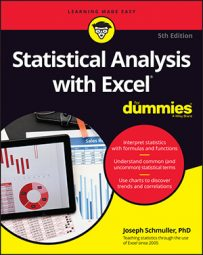##### Statistical Analysis with Excel For DummiesExcel’s ANOVA tool does not provide a built-in facility for carrying out planned (or unplanned) comparisons among the means. With a little ingenuity, however, you can use the Excel worksheet function `SUMPRODUCT` to do those comparisons.

The worksheet page with the ANOVA output is the launching pad for the planned comparisons. Here, you will be taken through one planned comparison — the mean of Method 1 versus the mean of Method 2.

Begin by creating columns that hold important information for the comparisons. The comparison coefficients are put in column J, the squares of those coefficients in column K, and the reciprocal of each sample size (1/n) in column L.

A few rows below those cells, you can put t-test-related information — the t-test numerator, the denominator, and the value of t. You can use separate cells for the numerator and denominator to simplify the formulas. You can put them together in one big formula and just have a cell for t, but it’s hard to keep track of everything.

`SUMPRODUCT` takes arrays of cells, multiplies the numbers in the corresponding cells, and sums the products. (This function is on the Math & Trig Functions menu, not the Statistical Functions menu.) You use `SUMPRODUCT` to multiply each coefficient by each sample mean and then add the products. That result was stored in K11. That’s the numerator for the planned comparison t-test. The formula for K11 is

`=SUMPRODUCT(J5:J7,D5:D7)`

The array J5:J7 holds the comparison coefficients, and D5:D7 holds the sample means.

K12 holds the denominator. K12 was selected in the image above so that you could see its formula in the Formula bar:

`=SQRT(D13*(SUMPRODUCT(K5:K7,L5:L7)))`

D13 has the MSW. `SUMPRODUCT` multiplies the squared coefficients in K5:K7 by the reciprocals of the sample sizes in L5:L7 and sums the products. `SQRT` takes the square root of the whole thing.

K13 holds the value for t. That’s just K11 divided by K12.

K14 presents the P-value for t — the proportion of area that t cuts off in the upper tail of the t-distribution with df = 24. The formula for that cell is

`=T.DIST.RT(K13,C13)`

The arguments are the calculated t (in K13) and the degrees of freedom for MSW (in C13).

If you change the coefficients in J5:J7, you instantaneously create and complete another comparison.

In fact, you can do that right now with Scheffé's post hoc comparison. That one, in this example, compares the mean of Method 1 with the mean of Method 3. This image shows the extra information for this test, starting a couple of rows below the t-test.

Cell K16 holds F, the square of the t value in K13. K17 has F', the product of C12 and G12 . K16 is greater than K17, so reject H0 for this comparison.